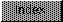```

mathffp.library/SPAbs
mathffp.library/SPCeil
mathffp.library/SPCmp
mathffp.library/SPDiv
mathffp.library/SPFix
mathffp.library/SPFloor
mathffp.library/SPFlt
mathffp.library/SPMul
mathffp.library/SPNeg
mathffp.library/SPSub
mathffp.library/SPTst
mathffp.library/SPAbs                                   mathffp.library/SPAbs

NAME
SPAbs -- Obtain the absolute value of the fast floating point number.

SYNOPSIS
fnum2 = SPAbs(fnum1)
D0	      D0

float SPAbs(float fnum1);

FUNCTION
Accepts a floating point number and returns the absolute value of
said number.

INPUTS
fnum1 	- floating point number.

RESULT
fnum2 	- floating point absolute value of fnum1.

BUGS
None

NAME

SYNOPSIS
D0	      D1     D0

FUNCTION
Accepts two floating point numbers and returns the arithmetic
sum of said numbers.

INPUTS
fnum1 	- floating point number to add.
fnum2 	- other floating point number to add.

RESULT
fnum3 	- floating point number, sum of fnum1 and fnum2.

BUGS
None.

mathffp.library/SPCeil                                 mathffp.library/SPCeil

NAME
SPCeil -- Compute Ceil function of a number.

SYNOPSIS
x = SPCeil(y)
D0         D0

float SPCeil(float y);

FUNCTION
Calculate the least integer greater than or equal to x and return it.
This identity is true.  Ceil(x) = -Floor(-x).

INPUTS
y 	- Motorola Fast Floating Point Format Number.

RESULT
x 	- Motorola Fast Floating Point Format Number.

BUGS
None.

SPFloor()

mathffp.library/SPCmp                                   mathffp.library/SPCmp

NAME
SPCmp -- Compares two floating point numbers.

SYNOPSIS
result = SPCmp(fnum1, fnum2)
D0               D0     D1

int SPCmp(float fnum1, float fnum2);

FUNCTION

Accepts two floating point numbers and returns the condition
codes set to indicate the result of said comparison.  Additionally,
the integer functional result is returned to indicate the result
of said comparison.

INPUTS
fnum1 	- floating point number.
fnum2 	- floating point number.

RESULT
Condition codes set to reflect the following branches:

GT - fnum2 >  fnum1
GE - fnum2 >= fnum1
EQ - fnum2 =  fnum1
NE - fnum2 != fnum1
LT - fnum2 <  fnum1
LE - fnum2 <= fnum1

Integer functional result as:

+1 => fnum1 > fnum2
-1 => fnum1 < fnum2
0 => fnum1 = fnum2

BUGS
None.

mathffp.library/SPDiv                                   mathffp.library/SPDiv

NAME
SPDiv -- Divide two floating point numbers.

SYNOPSIS
fnum3 = SPDiv(fnum1, fnum2)
D0	      D1     D0

float SPDiv(float fnum1, float fnum2);

FUNCTION
Accepts two floating point numbers and returns the arithmetic
division of said numbers.

INPUTS
fnum1 	- floating point number.
fnum2	- floating point number.

RESULT

fnum3 	- floating point number.

BUGS
None.

mathffp.library/SPFix                                   mathffp.library/SPFix

NAME
SPFix -- Convert fast floating point number to integer.

SYNOPSIS
inum = SPFix(fnum)
D0	     D0

int SPFix(float fnum);

FUNCTION
Accepts a floating point number and returns the truncated
integer portion of said number.

INPUTS

fnum 	- floating point number.

RESULT

inum 	- signed integer number.

BUGS
None.

mathffp.library/SPFloor                               mathffp.library/SPFloor

NAME
SPFloor -- compute Floor function of a number.

SYNOPSIS
x = SPFloor(y)
D0 	    D0

float SPFloor(float y);

FUNCTION
Calculate the largest integer less than or equal to x and return it.

INPUTS
y 	- Motorola Fast Floating Point number.

RESULT
x 	- Motorola Fast Floating Point number.

BUGS
None.

SPCeil()

mathffp.library/SPFlt                                   mathffp.library/SPFlt

NAME
SPFlt -- Convert integer number to fast floating point.

SYNOPSIS
fnum = SPFlt(inum)
D0	     D0

float SPFlt(int inum);

FUNCTION
Accepts an integer and returns the converted
floating point result of said number.

INPUTS
inum 	- signed integer number

RESULT
fnum 	- floating point number

BUGS
None.

mathffp.library/SPMul                                   mathffp.library/SPMul

NAME
SPMul -- Multiply two floating point numbers.

SYNOPSIS
fnum3 = SPMul(fnum1, fnum2)
D0	      D1     D0

float SPMul(float fnum1, float fnum2);

FUNCTION
Accepts two floating point numbers and returns the arithmetic
multiplication of said numbers.

INPUTS
fnum1 	- floating point number
fnum2 	- floating point number

RESULT
fnum3 	- floating point number

BUGS
None

mathffp.library/SPNeg                                   mathffp.library/SPNeg

NAME
SPNeg -- Negate the supplied floating point number.

SYNOPSIS
fnum2 = SPNeg(fnum1)
D0	      D0

float SPNeg(float fnum1);

FUNCTION
Accepts a floating point number and returns the value
of said number after having been subtracted from 0.0.

INPUTS
fnum1 	- floating point number.

RESULT
fnum2 	- floating point negation of fnum1.

BUGS
None

mathffp.library/SPSub                                   mathffp.library/SPSub

NAME
SPSub -- Subtract two floating point numbers.

SYNOPSIS
fnum3 = SPSub(fnum1, fnum2)
D0	      D1     D0

float SPSub(float fnum1, float fnum2);

FUNCTION
Accepts two floating point numbers and returns the arithmetic
subtraction of said numbers.

INPUTS
fnum1 	- floating point number.
fnum2 	- floating point number.

RESULT
fnum3 	- floating point number.

BUGS
None.

mathffp.library/SPTst                                   mathffp.library/SPTst

NAME
SPTst - Compares a float against zero (0.0).

SYNOPSIS
result = SPTst(fnum)
D0	       D1

int SPTst(float fnum);

FUNCTION
Accepts a floating point number and returns the condition
codes set to indicate the result of a comparison against
the value of zero (0.0).  Additionally, the integer functional
result is returned.

INPUTS
fnum 	- floating point number.

RESULT
Condition codes set to reflect the following branches:

EQ - fnum =  0.0
NE - fnum != 0.0
PL - fnum >= 0.0
MI - fnum <  0.0

Integer functional result as:

+1 => fnum > 0.0
-1 => fnum < 0.0
0 => fnum = 0.0

BUGS
None.